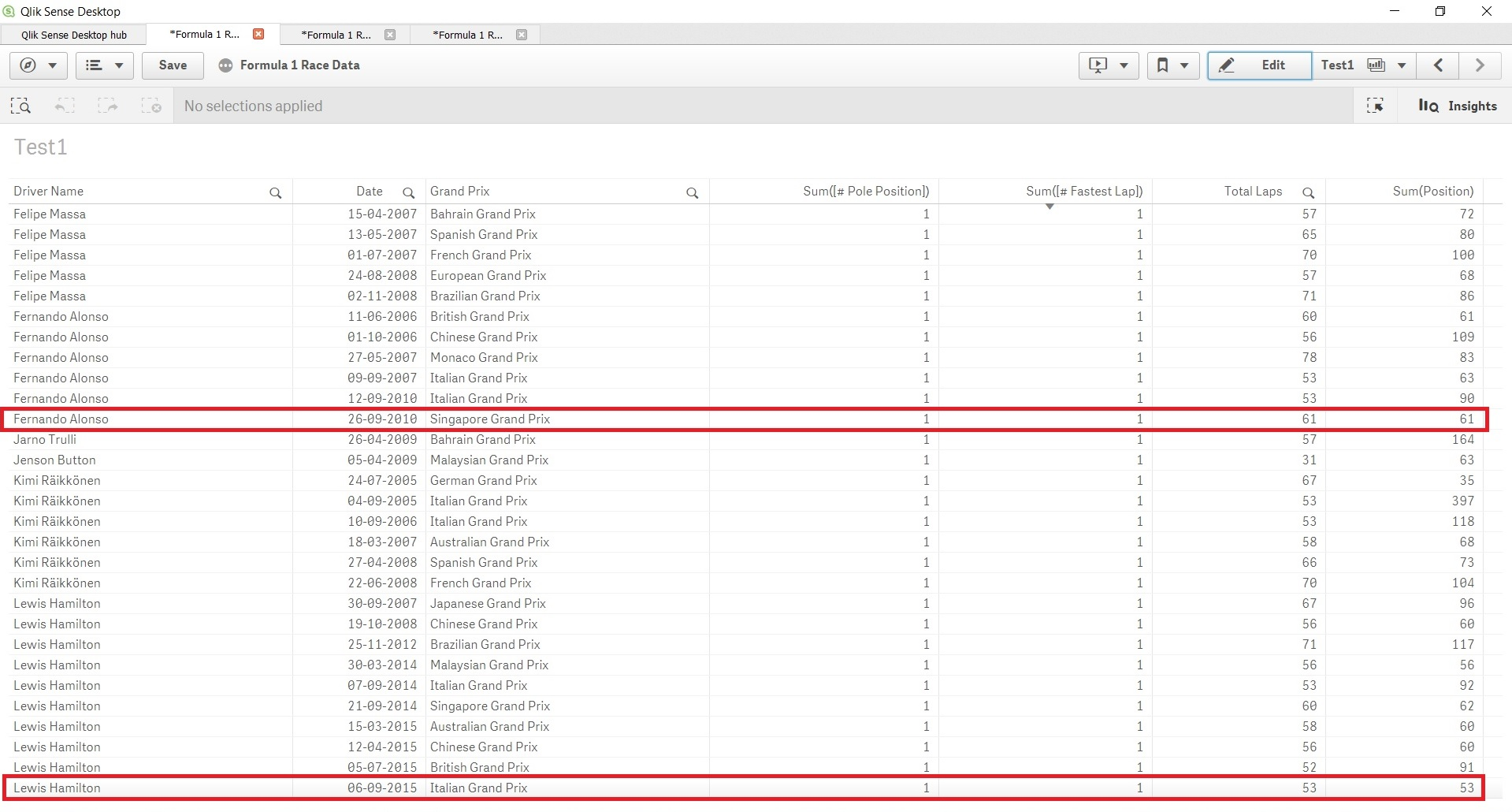# Qlik Sense App Development

Discussion board where members can learn more about Qlik Sense App Development and Usage.Partner

## Set Analysis - Field equal to another field

Hi all,

I am analyzing Formula 1 data and I would like to calculate the number of 'Grand Slam' wins by racing drivers. A racing driver earns a 'Grand Slam' when he starts at pole position, gets the fastest lap during the official race and drives the whole race at the first position.

For example, the picture below shows that Fernando Alonso and Lewis Hamilton have earned a Grand Slam, while both starts at pole position, gets the fastest lap and the total laps are equal to the sum of position (the position field exists of the rank of the racing driver per lap).

I am using the following expression, but unfortunately it is not working (shows only 0's):

Count({\$<[Starting Grid] = {1}, [# Fastest Lap] = {1}, [Total Laps] = {\$(=Sum(Position))}>}[End Ranking])

Does anybody know what I am doing wrong?

Thanks in advance! 🙂1 Solution

Accepted SolutionsMVP

## Re: Set Analysis - Field equal to another field

You can do an expression search on a field with a different syntax, but you need to apply the expression search on a field that uniquely identify the combination of driver and race.

I don't know your data, assuming that your data contains only driver that won the race, you could for example use the Date to identify a single race (and there will be only one driver per race). Or, create a combined key made of single race event and driver in your script.

Then:

Count({\$<[Starting Grid] = {1}, [# Fastest Lap] = {1}, CombinedKeyField = {"=Only([Total Laps])=Sum(Position)"}>}[End Ranking])

or with the assumption above

Count({\$<[Starting Grid] = {1}, [# Fastest Lap] = {1}, Date = {"=Only([Total Laps])=Sum(Position)"}>}[End Ranking])MVP

## Re: Set Analysis - Field equal to another field

You can do an expression search on a field with a different syntax, but you need to apply the expression search on a field that uniquely identify the combination of driver and race.

I don't know your data, assuming that your data contains only driver that won the race, you could for example use the Date to identify a single race (and there will be only one driver per race). Or, create a combined key made of single race event and driver in your script.

Then:

Count({\$<[Starting Grid] = {1}, [# Fastest Lap] = {1}, CombinedKeyField = {"=Only([Total Laps])=Sum(Position)"}>}[End Ranking])

or with the assumption above

Count({\$<[Starting Grid] = {1}, [# Fastest Lap] = {1}, Date = {"=Only([Total Laps])=Sum(Position)"}>}[End Ranking])